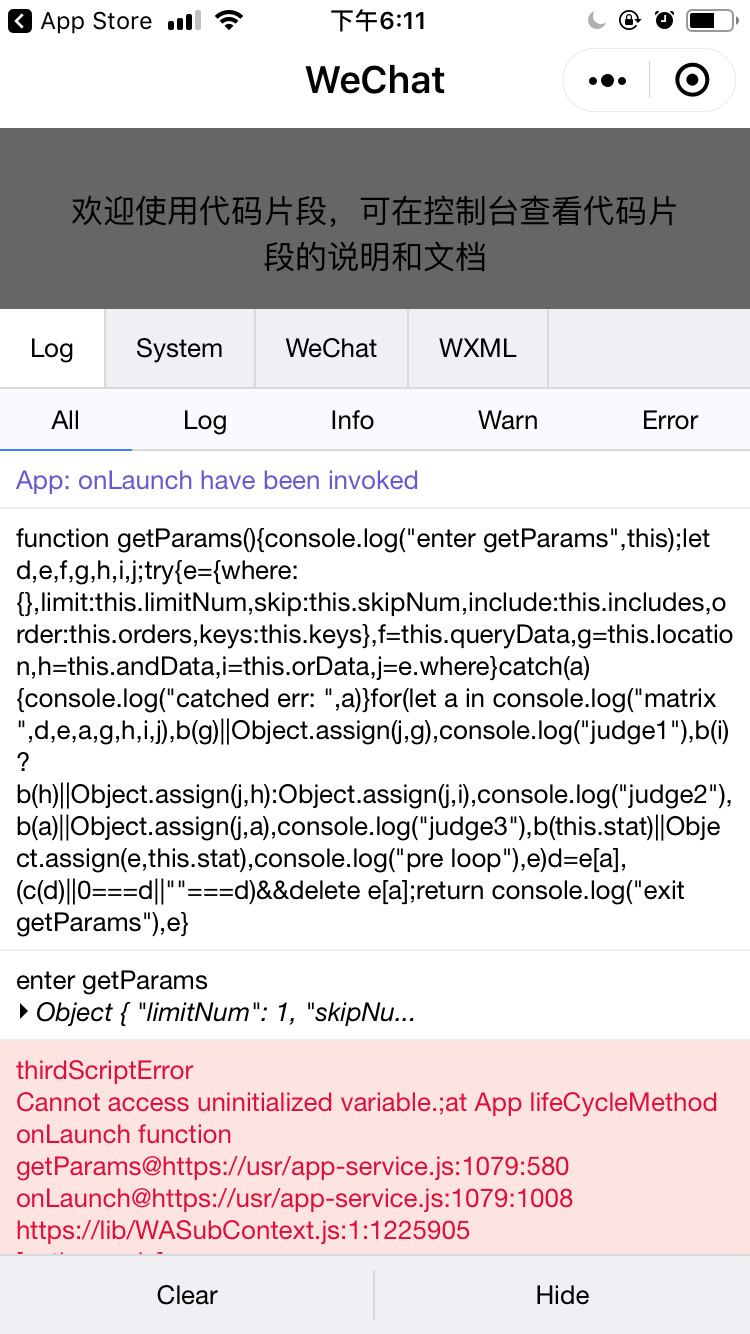# 置顶ES6语法+代码混淆导致声明错误精选热门

 `function` `test(){``  ``let obj = {``      ``test1: 1,``      ``test2: 2,``      ``test3: 3,``      ``test4: 4,``      ``test5: 5``    ``},``    ``tmp = ``""` `+ Date.now();` `  ``console.log(``"ts: "``, tmp);` `  ``for` `(let tmp ``in` `obj) {``    ``if` `(tmp) {``      ``console.log(``"有效key："``, tmp);``    ``}``  ``}``}`

 `function` `a() {``    ``let a = {``        ``test1: 1,``        ``test2: 2``    ``},``    ``b = ``""` `+ Date.now();` `    ``for` `(let b ``in` `console.log(``"ts: "``, b), a)``      ``b && console.log(``"\u6709\u6548key\uFF1A"``, b)``}`

iOS:1 个回答